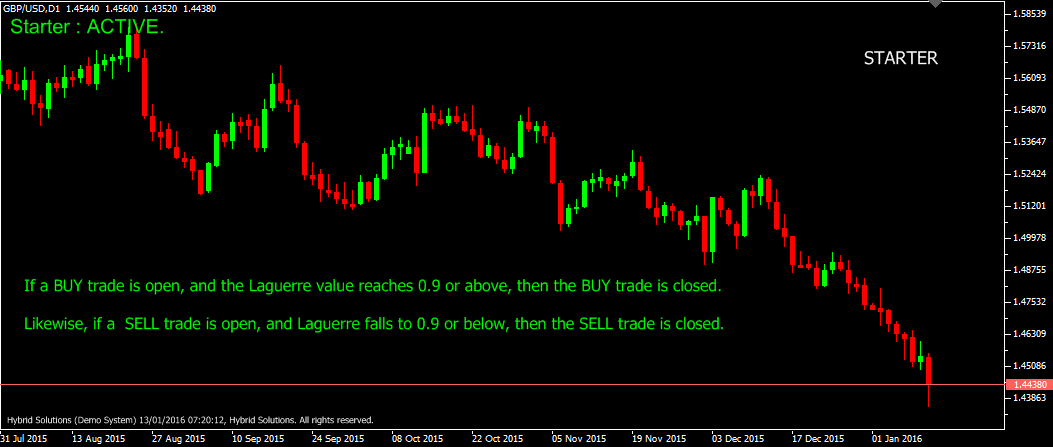# Starter

Free

Developed By: NeeduVerdeSolution

In stock

Starter is a powerful client side VertexFX Expert Advisor script that is based on Laguerre indicator, Commodity Channel Index (CCI) and Exponential Moving Average (EMA). If the Laguerre value is 0, and the CCI value is below -5 and the value of the EMA (of Median price) is greater than the previous candle value of the EMA, then a BUY trade is initiated. If the Laguerre value is 1, and the CCI value is above 5, and the value of the EMA (of Median price) is less than the previous candle value of the EMA, then a SELL trade is initiated.

SKU: 941 Categories: ,Starter is a powerful client side VertexFX Expert Advisor script that is based on Laguerre indicator, Commodity Channel Index (CCI) and Exponential Moving Average (EMA). If the Laguerre value is 0, and the CCI value is below -5 and the value of the EMA (of Median price) is greater than the previous candle value of the EMA, then a BUY trade is initiated. If the Laguerre value is 1, and the CCI value is above 5, and the value of the EMA (of Median price) is less than the previous candle value of the EMA, then a SELL trade is initiated.

If an open trade reaches a profit of TAKEPROFIT_POINTS points, then the trade is closed. If a BUY trade is open, and the Laguerre value reaches 0.9 or above, then the BUY trade is closed. Likewise, if a  SELL trade is open, and Laguerre falls to 0.9 or below, then the SELL trade is closed.

– MA PERIOD: The period used to calculate the Exponential Moving Average (EMA) of the median price.
– CCI PERIOD: The period over which the CCI indicator value is calculated.
– TAKE PROFIT POINTS: The take-profit in points of each trade. This script does not place the take-profit with the broker. It closes the position when the open profit reaches this value.
– LOT SIZE: The lot size of each trade opened by this script.
– MAGIC: The unique number to identify trades generated by this script.
– POINT DEFAULT: The default value of a point. The script uses this value if it is unable to detect the default value of a point.
– GAMMA: The smoothing factor used to calculate the Laguerre indicator.